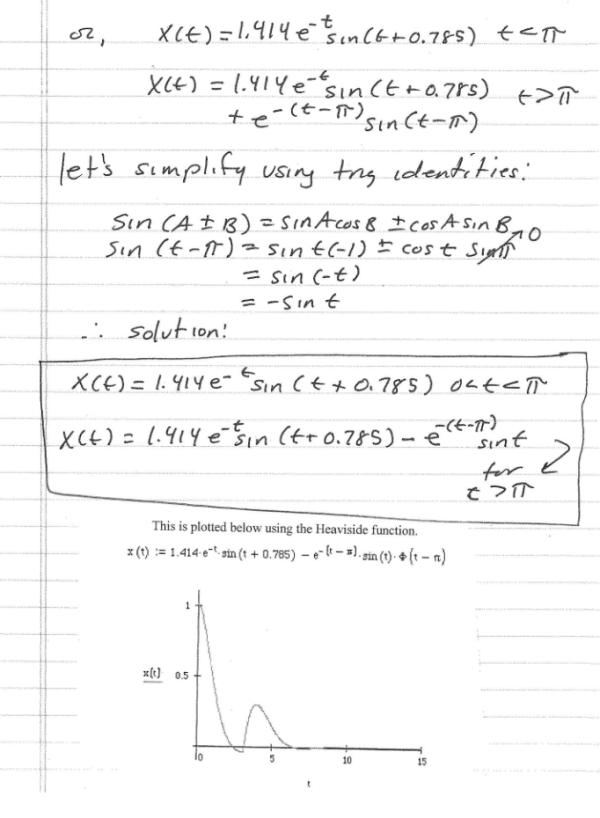Calculate the solution to xdoubledot + 2xdot + 2x = t-pi for x(0)=1 and xdot(0)=1, and plot the response.Calculate the solution to xdoubledot + 2xdot + 2x = t-pi for x(0)=1 and xdot(0)=1, and plot the response.

Vibrations Page 1 vibrations vibrations vibrations vibrations vibrations vibrations vibrations Vibrations Page 2